Want to share your content on R-bloggers? click here if you have a blog, or here if you don't.Many of you might have heard of the concept “Wisdom of the Crowd”: when many people independently guess some quantity, e.g. the number of marbles in a jar glass, the average of their guesses is often pretty accurate – even though many of the guesses are totally off.

The same principle is at work in so called ensemble methods, like bagging and boosting. If you want to know more about boosting and how to turn pseudocode of a scientific paper into valid R code read on…

We start from an original paper of one of the authors of the first practical boosting algorithm, i.e. AdaBoost: Robert E. Schapire: Explaining AdaBoost. The first sentence of the introduction gives the big idea:

Boosting is an approach to machine learning based on the idea of creating a highly accurate prediction rule by combining many relatively weak and inaccurate rules.

The second page gives the pseudocode of Adaboost…:

Given: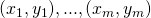where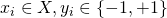.
Initialize:for.
For:

• Train weak learner using distribution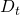.
• Get weak hypothesis:.
• Aim: selectwith low weighted error:• Choose.
• Update, for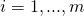: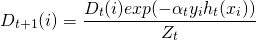whereis a normalization factor (chosen so thatwill be a distribution).

Output the final hypothesis: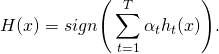… with some explanation:

[…] we are given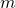labeled training exampleswhere theare in some domain, and the labels. On each round, a distributionis computed as in the figure over thetraining examples, and a given weak learner or weak learning algorithm is applied to find a weak hypothesis:, where the aim of the weak learner is to find a weak hypothesis with low weighted error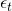relative to. The final or combined hypothesis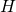computes the sign of a weighted combination of weak hypothesesThis is equivalent to saying thatis computed as a weighted majority vote of the weak hypotheseswhere each is assigned weight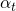. ([…] we use the terms “hypothesis” and “classifier” interchangeably.)

So, AdaBoost is adaptive in the sense that subsequent weak learners are tweaked in favor of those instances misclassified by previous ones. But to really understand what is going on my approach has always been that you haven’t really understood something before you didn’t build it yourself…

Perhaps you might want to try to translate the pseudocode into R code before reading on… (to increase your motivation I frankly admit that I also had some errors in my first implementation… which provides a good example of how strong the R community is because I posted it on stackoverflow and got a perfect answer two hours later: What is wrong with my implementation of AdaBoost?

Anyway, here is my implementation (the data can be found here: http://freakonometrics.free.fr/myocarde.csv):

library(rpart)
library(OneR)

maxdepth <- 1
T <- 100 # number of rounds

# Given: (x_1, y_1),...,(x_m, y_m) where x_i element of X, y_i element of {-1, +1}
y <- (myocarde[ , "PRONO"] == "SURVIE") * 2 - 1
x <- myocarde[ , 1:7]
m <- nrow(x)
data <- data.frame(x, y)

# Initialize: D_1(i) = 1/m for i = 1,...,m
D <- rep(1/m, m)

H <- replicate(T, list())
a <- vector(mode = "numeric", T)
set.seed(123)

# For t = 1,...,T
for(t in 1:T) {
# Train weak learner using distribution D_t
# Get weak hypothesis h_t: X -> {-1, +1}
H[[t]] <- rpart(y ~., data = data, weights = D, maxdepth = maxdepth, method = "class")
# Aim: select h_t with low weighted error: e_t = Pr_i~D_t[h_t(x_i) != y_i]
h <- predict(H[[t]], x, type = "class")
e <- sum((h!=y) * D)
# Choose a_t = 0.5 * log((1-e) / e)
a[t] <- 0.5 * log((1-e) / e)
# Update for i = 1,...,m: D_t+1(i) = (D_t(i) * exp(-a_t * y_i * h_t(x_i))) / Z_t
# where Z_t is a normalization factor (chosen so that Dt+1 will be a distribution)
D <- D * exp(-a[t] * y * as.numeric(as.character(h)))
D <- D / sum(D)
}

# Output the final hypothesis: H(x) = sign(sum of a_t * h_t(x) for t=1 to T)
newdata <- x
H_x <- sapply(H, function(x) as.numeric(as.character(predict(x, newdata = newdata, type = "class"))))
H_x <- t(a * t(H_x))
pred <- sign(rowSums(H_x))
eval_model(pred, y)
##
## Confusion matrix (absolute):
##           Actual
## Prediction -1  1 Sum
##        -1  29  0  29
##        1    0 42  42
##        Sum 29 42  71
##
## Confusion matrix (relative):
##           Actual
## Prediction   -1    1  Sum
##        -1  0.41 0.00 0.41
##        1   0.00 0.59 0.59
##        Sum 0.41 0.59 1.00
##
## Accuracy:
## 1 (71/71)
##
## Error rate:
## 0 (0/71)
##
## Error rate reduction (vs. base rate):
## 1 (p-value < 2.2e-16)


Let’s compare this with the result from the package JOUSBoost (on CRAN):

library(JOUSBoost)
## JOUSBoost 2.1.0

boost <- adaboost(as.matrix(x), y, tree_depth = maxdepth, n_rounds = T)
pred <- predict(boost, x)
eval_model(pred, y)
##
## Confusion matrix (absolute):
##           Actual
## Prediction -1  1 Sum
##        -1  29  0  29
##        1    0 42  42
##        Sum 29 42  71
##
## Confusion matrix (relative):
##           Actual
## Prediction   -1    1  Sum
##        -1  0.41 0.00 0.41
##        1   0.00 0.59 0.59
##        Sum 0.41 0.59 1.00
##
## Accuracy:
## 1 (71/71)
##
## Error rate:
## 0 (0/71)
##
## Error rate reduction (vs. base rate):
## 1 (p-value < 2.2e-16)


As you can see: zero errors as with my implementation. Two additional remarks are in order:

An accuracy of 100% hints at one of the problems of boosting: it is prone to overfitting (see also Learning Data Science: Modelling Basics).

The second problem is the lack of interpretability: whereas decision trees are normally well interpretable ensembles of them are not. This is also known under the name Accuracy-Interpretability Trade-Off (another often used ensemble method is random forests, see also here: Learning Data Science: Predicting Income Brackets).

Hope that this post was helpful for you to understand the widely used boosting methodology better and to see how you can get from pseudocode to valid R code. If you have any questions or feedback please let me know in the comments – Thank you and stay tuned!